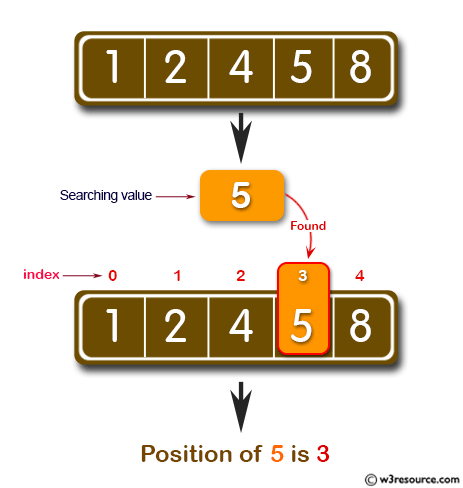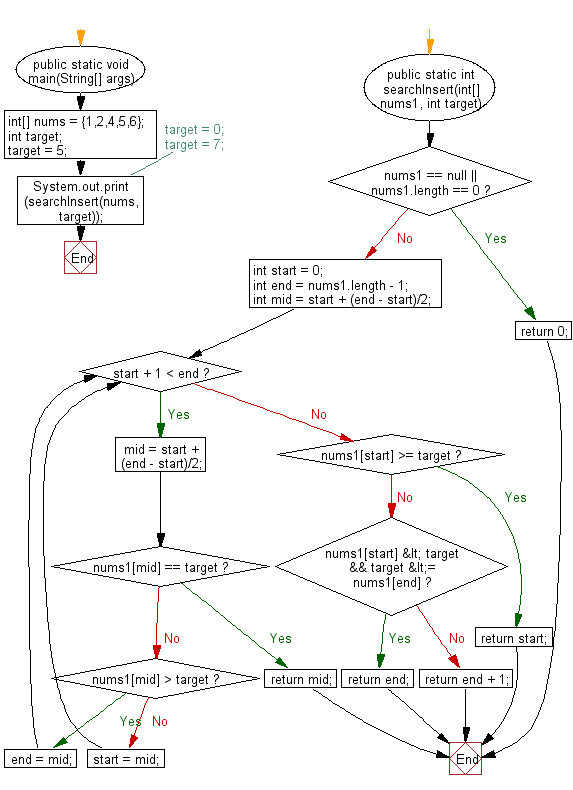﻿ Java exercises: Find the index of a value in a sorted array - w3resource# Java Exercises: Find the index of a value in a sorted array.

## Java Basic: Exercise-124 with Solution

Write a Java program to find the index of a value in a sorted array. If the value does not find return the index where it would be if it were inserted in order. Example:
[1, 2, 4, 5, 6] 5(target) -> 3(index)
[1, 2, 4, 5, 6] 0(target) -> 0(index)
[1, 2, 4, 5, 6] 7(target) -> 5(index)

Pictorial Presentation:Sample Solution:

Java Code:

``````import java.util.*;
public class Main {
public static void main(String[] args) {
int[] nums = {1,2,4,5,6};
int target;
target = 5;
// target = 0;
// target = 7;
System.out.print(searchInsert(nums, target));
}
public static int searchInsert(int[] nums1, int target) {
if (nums1 == null || nums1.length == 0) {
return 0;
}
int start = 0;
int end = nums1.length - 1;
int mid = start + (end - start)/2;

while (start + 1 < end) {
mid = start + (end - start)/2;
if (nums1[mid] == target) {
return mid;
} else if (nums1[mid] > target) {
end = mid;
} else {
start = mid;
}
}

if (nums1[start] >= target) {
return start;
} else if (nums1[start] < target && target <= nums1[end]) {
return end;
} else {
return end + 1;
}
}
}
```
```

Sample Output:

```3
```

Flowchart:Java Code Editor:

What is the difficulty level of this exercise?

Test your Programming skills with w3resource's quiz.

﻿

## Java: Tips of the Day

countOccurrences

Counts the occurrences of a value in an array.

Use Arrays.stream().filter().count() to count total number of values that equals the specified value.

```public static long countOccurrences(int[] numbers, int value) {
return Arrays.stream(numbers)
.filter(number -> number == value)
.count();
}
```

Ref: https://bit.ly/3kCAgLb